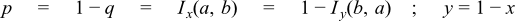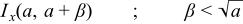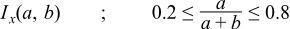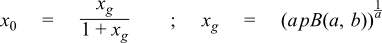#Boost C++ Libraries

...one of the most highly regarded and expertly designed C++ library projects in the world.

This is the documentation for an old version of boost. Click here for the latest Boost documentation.

#### The Incomplete Beta Function Inverses

```#include <boost/math/special_functions/beta.hpp>
```

```namespace boost{ namespace math{

template <class T1, class T2, class T3>
calculated-result-type ibeta_inv(T1 a, T2 b, T3 p);

template <class T1, class T2, class T3, class Policy>
calculated-result-type ibeta_inv(T1 a, T2 b, T3 p, const Policy&);

template <class T1, class T2, class T3, class T4>
calculated-result-type ibeta_inv(T1 a, T2 b, T3 p, T4* py);

template <class T1, class T2, class T3, class T4, class Policy>
calculated-result-type ibeta_inv(T1 a, T2 b, T3 p, T4* py, const Policy&);

template <class T1, class T2, class T3>
calculated-result-type ibetac_inv(T1 a, T2 b, T3 q);

template <class T1, class T2, class T3, class Policy>
calculated-result-type ibetac_inv(T1 a, T2 b, T3 q, const Policy&);

template <class T1, class T2, class T3, class T4>
calculated-result-type ibetac_inv(T1 a, T2 b, T3 q, T4* py);

template <class T1, class T2, class T3, class T4, class Policy>
calculated-result-type ibetac_inv(T1 a, T2 b, T3 q, T4* py, const Policy&);

template <class T1, class T2, class T3>
calculated-result-type ibeta_inva(T1 b, T2 x, T3 p);

template <class T1, class T2, class T3, class Policy>
calculated-result-type ibeta_inva(T1 b, T2 x, T3 p, const Policy&);

template <class T1, class T2, class T3>
calculated-result-type ibetac_inva(T1 b, T2 x, T3 q);

template <class T1, class T2, class T3, class Policy>
calculated-result-type ibetac_inva(T1 b, T2 x, T3 q, const Policy&);

template <class T1, class T2, class T3>
calculated-result-type ibeta_invb(T1 a, T2 x, T3 p);

template <class T1, class T2, class T3, class Policy>
calculated-result-type ibeta_invb(T1 a, T2 x, T3 p, const Policy&);

template <class T1, class T2, class T3>
calculated-result-type ibetac_invb(T1 a, T2 x, T3 q);

template <class T1, class T2, class T3, class Policy>
calculated-result-type ibetac_invb(T1 a, T2 x, T3 q, const Policy&);

}} // namespaces
```
##### Description

There are six incomplete beta function inverses which allow you solve for any of the three parameters to the incomplete beta, starting from either the result of the incomplete beta (p) or its complement (q).

The final Policy argument is optional and can be used to control the behaviour of the function: how it handles errors, what level of precision to use etc. Refer to the policy documentation for more details.

TipWhen people normally talk about the inverse of the incomplete beta function, they are talking about inverting on parameter x. These are implemented here as ibeta_inv and ibetac_inv, and are by far the most efficient of the inverses presented here. The inverses on the a and b parameters find use in some statistical applications, but have to be computed by rather brute force numerical techniques and are consequently several times slower. These are implemented here as ibeta_inva and ibeta_invb, and complement versions ibetac_inva and ibetac_invb.

The return type of these functions is computed using the result type calculation rules when called with arguments T1...TN of different types.

```template <class T1, class T2, class T3>
calculated-result-type ibeta_inv(T1 a, T2 b, T3 p);

template <class T1, class T2, class T3, class Policy>
calculated-result-type ibeta_inv(T1 a, T2 b, T3 p, const Policy&);

template <class T1, class T2, class T3, class T4>
calculated-result-type ibeta_inv(T1 a, T2 b, T3 p, T4* py);

template <class T1, class T2, class T3, class T4, class Policy>
calculated-result-type ibeta_inv(T1 a, T2 b, T3 p, T4* py, const Policy&);
```

Returns a value x such that: ```p = ibeta(a, b, x);``` and sets ```*py = 1 - x``` when the `py` parameter is provided and is non-null. Note that internally this function computes whichever is the smaller of `x` and `1-x`, and therefore the value assigned to `*py` is free from cancellation errors. That means that even if the function returns `1`, the value stored in `*py` may be non-zero, albeit very small.

Requires: a,b > 0 and 0 <= p <= 1.

The final Policy argument is optional and can be used to control the behaviour of the function: how it handles errors, what level of precision to use etc. Refer to the policy documentation for more details.

```template <class T1, class T2, class T3>
calculated-result-type ibetac_inv(T1 a, T2 b, T3 q);

template <class T1, class T2, class T3, class Policy>
calculated-result-type ibetac_inv(T1 a, T2 b, T3 q, const Policy&);

template <class T1, class T2, class T3, class T4>
calculated-result-type ibetac_inv(T1 a, T2 b, T3 q, T4* py);

template <class T1, class T2, class T3, class T4, class Policy>
calculated-result-type ibetac_inv(T1 a, T2 b, T3 q, T4* py, const Policy&);
```

Returns a value x such that: ```q = ibetac(a, b, x);``` and sets ```*py = 1 - x``` when the `py` parameter is provided and is non-null. Note that internally this function computes whichever is the smaller of `x` and `1-x`, and therefore the value assigned to `*py` is free from cancellation errors. That means that even if the function returns `1`, the value stored in `*py` may be non-zero, albeit very small.

Requires: a,b > 0 and 0 <= q <= 1.

The final Policy argument is optional and can be used to control the behaviour of the function: how it handles errors, what level of precision to use etc. Refer to the policy documentation for more details.

```template <class T1, class T2, class T3>
calculated-result-type ibeta_inva(T1 b, T2 x, T3 p);

template <class T1, class T2, class T3, class Policy>
calculated-result-type ibeta_inva(T1 b, T2 x, T3 p, const Policy&);
```

Returns a value a such that: ```p = ibeta(a, b, x);```

Requires: b > 0, 0 < x < 1 and 0 <= p <= 1.

The final Policy argument is optional and can be used to control the behaviour of the function: how it handles errors, what level of precision to use etc. Refer to the policy documentation for more details.

```template <class T1, class T2, class T3>
calculated-result-type ibetac_inva(T1 b, T2 x, T3 p);

template <class T1, class T2, class T3, class Policy>
calculated-result-type ibetac_inva(T1 b, T2 x, T3 p, const Policy&);
```

Returns a value a such that: ```q = ibetac(a, b, x);```

Requires: b > 0, 0 < x < 1 and 0 <= q <= 1.

The final Policy argument is optional and can be used to control the behaviour of the function: how it handles errors, what level of precision to use etc. Refer to the policy documentation for more details.

```template <class T1, class T2, class T3>
calculated-result-type ibeta_invb(T1 b, T2 x, T3 p);

template <class T1, class T2, class T3, class Policy>
calculated-result-type ibeta_invb(T1 b, T2 x, T3 p, const Policy&);
```

Returns a value b such that: ```p = ibeta(a, b, x);```

Requires: a > 0, 0 < x < 1 and 0 <= p <= 1.

The final Policy argument is optional and can be used to control the behaviour of the function: how it handles errors, what level of precision to use etc. Refer to the policy documentation for more details.

```template <class T1, class T2, class T3>
calculated-result-type ibetac_invb(T1 b, T2 x, T3 p);

template <class T1, class T2, class T3, class Policy>
calculated-result-type ibetac_invb(T1 b, T2 x, T3 p, const Policy&);
```

Returns a value b such that: ```q = ibetac(a, b, x);```

Requires: a > 0, 0 < x < 1 and 0 <= q <= 1.

The final Policy argument is optional and can be used to control the behaviour of the function: how it handles errors, what level of precision to use etc. Refer to the policy documentation for more details.

##### Accuracy

The accuracy of these functions should closely follow that of the regular forward incomplete beta functions. However, note that in some parts of their domain, these functions can be extremely sensitive to changes in input, particularly when the argument p (or it's complement q) is very close to `0` or `1`.

##### Testing

There are two sets of tests:

• Basic sanity checks attempt to "round-trip" from a, b and x to p or q and back again. These tests have quite generous tolerances: in general both the incomplete beta and its inverses change so rapidly, that round tripping to more than a couple of significant digits isn't possible. This is especially true when p or q is very near one: in this case there isn't enough "information content" in the input to the inverse function to get back where you started.
• Accuracy checks using high precision test values. These measure the accuracy of the result, given exact input values.
##### Implementation of ibeta_inv and ibetac_inv

These two functions share a common implementation.

First an initial approximation to x is computed then the last few bits are cleaned up using Halley iteration. The iteration limit is set to 1/2 of the number of bits in T, which by experiment is sufficient to ensure that the inverses are at least as accurate as the normal incomplete beta functions. Up to 5 iterations may be required in extreme cases, although normally only one or two are required. Further, the number of iterations required decreases with increasing a and b (which generally form the more important use cases).

The initial guesses used for iteration are obtained as follows:

Firstly recall that:We may wish to start from either p or q, and to calculate either x or y. In addition at any stage we can exchange a for b, p for q, and x for y if it results in a more manageable problem.

For `a+b >= 5` the initial guess is computed using the methods described in:

Asymptotic Inversion of the Incomplete Beta Function, by N. M. Temme. Journal of Computational and Applied Mathematics 41 (1992) 145-157.

The nearly symmetrical case (section 2 of the paper) is used forand involves solving the inverse error function first. The method is accurate to at least 2 decimal digits when `a = 5` rising to at least 8 digits when `a = 105`.

The general error function case (section 3 of the paper) is used forand again expresses the inverse incomplete beta in terms of the inverse of the error function. The method is accurate to at least 2 decimal digits when `a+b = 5` rising to 11 digits when ```a+b = 105```. However, when the result is expected to be very small, and when a+b is also small, then its accuracy tails off, in this case when p1/a < 0.0025 then it is better to use the following as an initial estimate:Finally the for all other cases where ```a+b > 5``` the method of section 4 of the paper is used. This expresses the inverse incomplete beta in terms of the inverse of the incomplete gamma function, and is therefore significantly more expensive to compute than the other cases. However the method is accurate to at least 3 decimal digits when `a = 5` rising to at least 10 digits when `a = 105`. This method is limited to a > b, and therefore we need to perform an exchange a for b, p for q and x for y when this is not the case. In addition when p is close to 1 the method is inaccurate should we actually want y rather than x as output. Therefore when q is small (`q1/p < 10-3`) we use:which is both cheaper to compute than the full method, and a more accurate estimate on q.

When a and b are both small there is a distinct lack of information in the literature on how to proceed. I am extremely grateful to Prof Nico Temme who provided the following information with a great deal of patience and explanation on his part. Any errors that follow are entirely my own, and not Prof Temme's.

When a and b are both less than 1, then there is a point of inflection in the incomplete beta at point ```xs = (1 - a) / (2 - a - b)```. Therefore if `p > Ix(a,b)` we swap a for b, p for q and x for y, so that now we always look for a point x below the point of inflection `xs`, and on a convex curve. An initial estimate for x is made with:which is provably below the true value for x: Newton iteration will therefore smoothly converge on x without problems caused by overshooting etc.

When a and b are both greater than 1, but a+b is too small to use the other methods mentioned above, we proceed as follows. Observe that there is a point of inflection in the incomplete beta at ```xs = (1 - a) / (2 - a - b)```. Therefore if `p > Ix(a,b)` we swap a for b, p for q and x for y, so that now we always look for a point x below the point of inflection `xs`, and on a concave curve. An initial estimate for x is made with:which can be improved somewhat to:when b and x are both small (I've used b < a and x < 0.2). This actually under-estimates x, which drops us on the wrong side of x for Newton iteration to converge monotonically. However, use of higher derivatives and Halley iteration keeps everything under control.

The final case to be considered if when one of a and b is less than or equal to 1, and the other greater that 1. Here, if b < a we swap a for b, p for q and x for y. Now the curve of the incomplete beta is convex with no points of inflection in [0,1]. For small p, x can be estimated usingwhich under-estimates x, and drops us on the right side of the true value for Newton iteration to converge monotonically. However, when p is large this can quite badly underestimate x. This is especially an issue when we really want to find y, in which case this method can be an arbitrary number of order of magnitudes out, leading to very poor convergence during iteration.

Things can be improved by considering the incomplete beta as a distorted quarter circle, and estimating y from:This doesn't guarantee that we will drop in on the right side of x for monotonic convergence, but it does get us close enough that Halley iteration rapidly converges on the true value.

##### Implementation of inverses on the a and b parameters

These four functions share a common implementation.

First an initial approximation is computed for a or b: where possible this uses a Cornish-Fisher expansion for the negative binomial distribution to get within around 1 of the result. However, when a or b are very small the Cornish Fisher expansion is not usable, in this case the initial approximation is chosen so that Ix(a, b) is near the middle of the range [0,1].

This initial guess is then used as a starting value for a generic root finding algorithm. The algorithm converges rapidly on the root once it has been bracketed, but bracketing the root may take several iterations. A better initial approximation for a or b would improve these functions quite substantially: currently 10-20 incomplete beta function invocations are required to find the root.

 Copyright © 2006-2010 John Maddock, Paul A. Bristow, Hubert Holin, Xiaogang Zhang, Bruno Lalande, Johan Råde, Gautam Sewani and Thijs van den Berg Distributed under the Boost Software License, Version 1.0. (See accompanying file LICENSE_1_0.txt or copy at http://www.boost.org/LICENSE_1_0.txt)